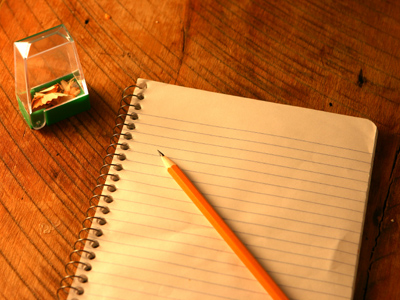A paper and pencil could be handy for this quiz.

# Letters for Numbers 3

In this series of 11-plus verbal reasoning quizzes letters stand for numbers. Work out the correct answer to each sum and choose the correct answer from the four choices available.

This quiz has some interesting facts included. Look for the number of doors to heaven and hell in the Islamic tradition!

Enjoy Letters for Numbers quiz 3 and see if you can get full marks using your powers of detection.

Please note that these questions assume you have not yet learned BODMAS, so if you have, please don't use BODMAS!

Example: What is the answer to the sum below, written as a letter?

A = 5, B = 14, C = 12, D = 13, E = 3
A + C – E = ?

A
B
C
E

Answer: The correct answer is B. If we translate all the letters into numbers, our new sum is 5 + 12 – 3, which = 14. 14 = B.
1.
What is the answer to the sum, written as a letter?

S = 63, O = 9, A = 2, P = 7
S ÷ O x A - P
S
O
A
P
If we translate all the letters into numbers, our new sum is 63 ÷ 9 x 2 - 7, which = 7. 7 = P
2.
What is the answer to the sum, written as a letter?

N = 12, E = 6, W = 4, S = 3
N x E ÷ S ÷ W
N
E
S
W
If we translate all the letters into numbers, our new sum is 12 x 6 ÷ 3 ÷ 4, which = 6. 6 = E. Interesting number alert! The number 6 is the smallest perfect number. A perfect number is the sum of its divisors. This means that all the numbers that will divide equally into it also add up to it. 1, 2 and 3 are the numbers which divide into 6, and they also add up to six. Perfect!
3.
What is the answer to the sum, written as a letter?

F = 64, O = 4, U = 19, R = 7
F ÷ O + R - U
F
O
U
R
If we translate all the letters into numbers, our new sum is 64 ÷ 4 + 7 - 19, which = 4. 4 = O. Interesting number alert! 64 is the highest two digit number which can be divided by 2, by 2 again, again and again right down to 1
4.
What is the answer to the sum, written as a letter?

F = 11, I = 16, V = 9, E = 8
F + I ÷ V + E
F
I
V
E
If we translate all the letters into numbers, our new sum is 11 + 16 ÷ 9 + 8, which = 11. 11 = F. Interesting number alert! The number 11 is the number of players in soccer, hockey and cricket!
5.
What is the answer to the sum, written as a letter?

T = 67, R = 60, A = 11, P = 10
T + P ÷ A + R
T
R
A
P
If we translate all the letters into numbers, our new sum is 67 + 10 ÷ 11 + 60, which = 67. 67 = T. Interesting number alert! 60 is the smallest number which can be divided by 1, 2, 3, 4, 5 and 6. Very handy!
6.
What is the answer to the sum, written as a letter?

Q = 19, U = 2, I = 17, T = 38
T - I + Q - U
Q
U
I
T
If we translate all the letters into numbers, our new sum is 38 - 17 + 19 - 2, which = 38. 38 = T
7.
What is the answer to the sum, written as a letter?

T = 30, I = 6, M = 3, E = 15
T ÷ I x M + E
T
I
M
E
If we translate all the letters into numbers, our new sum is 30 ÷ 6 x 3 + 15, which = 30. 30 = T
8.
What is the answer to the sum, written as a letter?

C = 14, U = 1, P = 7, S = 5
C ÷ P + S x U
C
U
P
S
If we translate all the letters into numbers, our new sum is 14 ÷ 7 + 5 x 1, which = 7. 7 = P. Interesting number alert! The number 7 has a special importance in many cultures. In the Islamic tradition, there are seven heavens and seven Earths. The numbers of fires in hell and the number of doors to both heaven and hell is also seven!
9.
What is the answer to the sum, written as a letter?

A = 43, R = 2, G = 34, H = 26
A - H x R
A
R
G
H
If we translate all the letters into numbers, our new sum is 43 - 26 x 2, which = 34. 34 = G
10.
What is the answer to the sum, written as a letter?

M = 44, I = 38, N = 2, X = 11
M ÷ X + N + I
M
I
N
X
If we translate all the letters into numbers, our new sum is 44 ÷ 11 + 2 + 38, which = 44. 44 = M. Well. You have completed this quiz. There is only one more Letters for Numbers quiz in the series, so you have completed 75% or three quarters of the series!
Author:  Stephen O'Hara